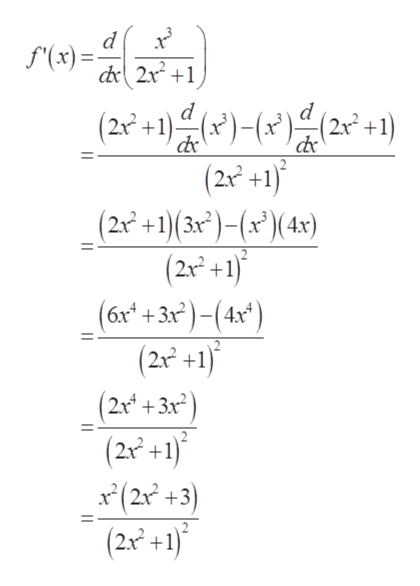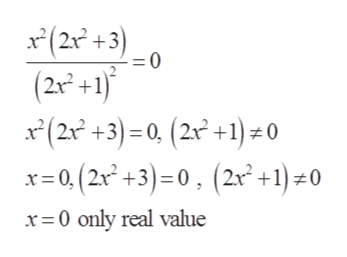Question
7 views

Can you help with this problem step by step?

check_circle

Step 1

Given function is

Step 2

For increasing and decreasing of function, derivate the above functionhelp_outlineImage Transcriptionclosed f'(x)= dr2x21 (2x+1))2) (2x2 +1) (22 +1)( 3x)-(x') (4x) (2x2+1) (6x +3x)-(4x) (2x +1) (2x+3x (2n +1) x(2x2 +3) (2n2+1) d fullscreen
Step 3

Critical points are the points where function is define...help_outlineImage Transcriptionclose(2x +3) (2x+1) x(22 +3)=0, (2x +1) - 0 x=0, (2x2 +3)=0, (2r +1) *0. =0 x 0 only real value fullscreen

### Want to see the full answer?

See Solution

#### Want to see this answer and more?

Solutions are written by subject experts who are available 24/7. Questions are typically answered within 1 hour.*

See Solution
*Response times may vary by subject and question.
Tagged in
MathCalculus

### Functions